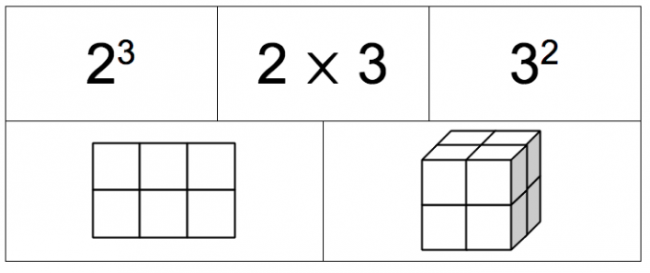# Cubes versus Squares

Preview:The goal of this task to provide students with a model for how cube numbers can be represented with cubes and square numbers can be represented with squares.

Connecting Representations
Big Idea:
Grade 8: Number represents, describes, and compares the quantities of ratios, rates, and percents.
Grade 8: Computational fluency and flexibility extend to operations with fractions.
Content:
perfect squares and cubes using colour tiles, pictures, or multi-link cubes building the number or using prime factorization
Curricular Competency:
Reasoning and analyzing: Develop mental math strategies and abilities to make sense of quantities
Understanding and solving: Develop, demonstrate, and apply mathematical understanding through play, inquiry, and problem solving
Understanding and solving: Visualize to explore mathematical concepts
Understanding and solving: Develop and use multiple strategies to engage in problem solving
Communicating and representing: Communicate mathematical thinking in many ways
Communicating and representing: Use mathematical vocabulary and language to contribute to mathematical discussions
Communicating and representing: Explain and justify mathematical ideas and decisions
Communicating and representing: Represent mathematical ideas in concrete, pictorial, and symbolic forms
Connecting and reflecting: Reflect on mathematical thinking